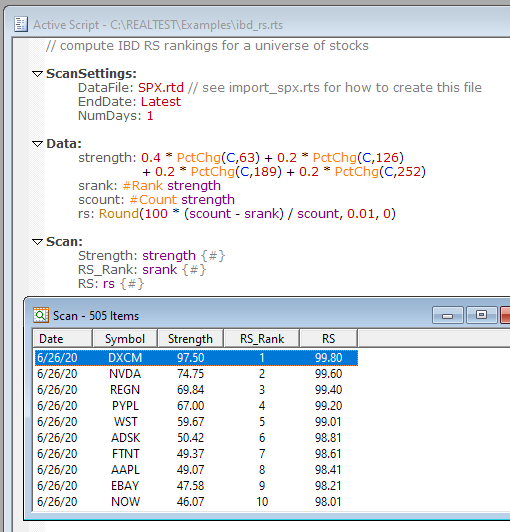Navigation: Realtest Script Language > Formula Syntax > Breadth Tags / Cross-Sectional Functions

RealTest makes it easy to specify and calculate cross-sectional or "breadth" functions in the Data Section of a script.

Whereas the usual rolling bar functions such as Avg, Sum, Highest, Lowest, etc. operate "vertically" (down a column of the same symbol for different dates), the breadth functions operate "horizontally" (across a row of the same date for different symbols).

The format of a data item that calculates a breadth function is:

name: #function formula

Name is the name of the data item, as usual.

Function is the specific breadth function to use.

Formula is the formula to calculate for each cell (a specific symbol on a specific date).

Only one breadth function can be used in a data item, and it must appear at the start of the formula for that item.

Any number of data items can have breadth tags, though, so if you need to perform a multi-level breadth calculation, just use multiple data items.

When the item is calculated for each date, the following things occur:

1.formula is calculated for every symbol with date for that date

2.function is calculated for the set of values produced by step 1

3.the result is stored as the value of the data item for every symbol for that date

Depending on which function is used, the end result may be the same for every symbol on a given date.

#Avg, #Count, #Highest, #Lowest, #Median, #StdDev and #Sum will, by definition, all produce the same answer for every cell in a row of data.

Don't worry, formula will still only be evaluated once per symbol per date, and function is only calculated once per date. The point is that this result is then separately stored for every symbol for that date. While this might sound silly, it actually simplifies other formulas that want to access this result, because they can simply refer to it in the context of their own current symbol.

#PercentRank and #Rank, on the other hand, will (by definition) produce a different answer for every cell in a row of data. In this case, formula is still only evaluated once per symbol per date, after which function is calculated by sorting the resulting array of values and then storing each symbol's ordinal (expressed as rank percent or rank number) as its data value for that date.

The example script ibd_rs.rts shows how to use this feature to calculate IBD-style "relative strength" ranking.

Here is how the S&P 500 components were ranked for IBD RS on 6/26/20:Note that if a breadth function formula result is nan (not a number -- unable to calculate) then that stock is not included in the ranking list (reducing the total count) and its rank value would be nan as well. Similarly, nan values would not be included in the value count for any breadth functions that use it (e.g. #Avg, #Median, #StdDev).